# Mathematics 1010 online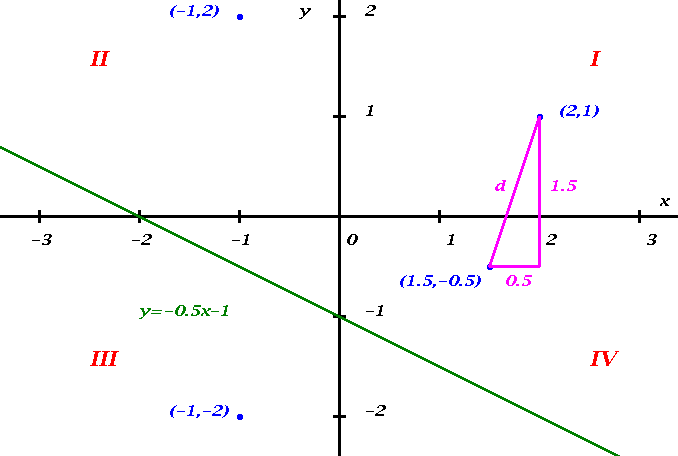## The Cartesian Coordinate System

You are actually familiar with Cartesian Coordinates, they are used to express addresses in Salt Lake City. The Cartesian Coordinate System (also called Rectangular Coordinate System ) is named after its inventor Renee Descartes (1596-1650). It greatly amplifies our problem solving ability by letting us think about algebraic problems geometrically, and about geometric problems algebraically.

### Axes

Consider the Figure above. The Cartesian Coordinate System consists of a vertical and a horizontal number line that intersect perpendicularly at their origins. The intersection is also called the origin of the coordinate system. The number lines are called the axes of the system. The word axes is the plural of the word axis. Thus there is a horizontal axis, and a vertical axis. The variable along the horizontal axes is commonly called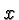, and that along the vertical axis is called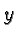. Other choices are possible, but we will use this particular choice almost universally in this class. Accordingly the horizontal axis is also called the-axis, and the vertical axis the-axis. Collectively the axes are also called the coordinate axes. By convention the horizontal axis points to the right, and the vertical axis points up.

### Coordinates

The location of a point can be described by two numbers that are obtained by projecting the point perpendicularly onto each of the coordinate axes. The projection onto the horizontal axis is the-coordinate, and the projection onto the vertical axis the-coordinate. A point is described by the two coordinates, separated by a comma and enclosed by parentheses, with the-coordinate coming first. The Figure above shows four points --specifically the points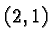,,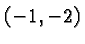, and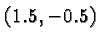-- and their representation as pairs of numbers, all drawn in blue.

Conversely, given two numbers, one being the-coordinate and the other the-coordinate, there is a unique point in the Cartesian Coordinates System that corresponds to that pair of numbers.

The two coordinate axes divide the plane into four parts. These are called quadrants, and they are numbered I, II, III, IV, starting with the Northeast quadrant and moving counterclockwise. The quadrants are indicated in red in the above Figure. We talk about the first, second, etc., quadrant.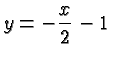drawn in green.

### The Distance between Two Points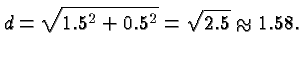Drawing a similar picture involving two general points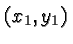andyou can see that the distance between these two points is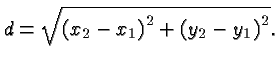Note that it does not matter which point you call point 1 and which point 2, and whether some of the coordinates involved are negative. If these facts are at all mysterious to you think about them carefully! In any case, don't memorize the above distance formula, it is a simple and obvious consequence of the Pythagorean Theorem, and if you think of it that way you'll always be able to write it down from scratch even if you don't "remember" it.